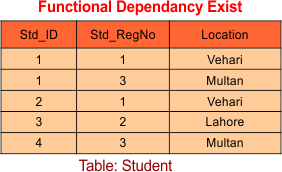Select Page

# Second Normal Form (2NF)

Before to learn 2NF, 3NF, BCNF, 4NF and 5NF you must know about

A table will be in 2NF if it follow the followings

1. Table should be in the First Normal form (1NF).

2. There should no  Partial Dependency in the relation, it means all the non-prime attributes should be fully functional dependent on candidate key.

Partial dependency: A part of candidate key is determining the non-prime attribute is called partial dependency. Suppose AB is the candidate key, if a part of candidate key (i.e. A) determines the non-prime attribute (i.e. X). Like A → X, then it is partial dependency.

3. Non-prime attribute never determines the non-prime attribute

## Question Point of View for 2NF

If following conditions exists then table will not be in 2NF

1. According to partial dependency, L.H.S should be the proper subset of Candidate key AND R.H.S should be non-prime attributes. (proper subset never be equal to actual candidate key)

2. Non-prime attribute determining non-prime attribute

Note: Prime attribute can determine Prime attribute, Prime can determine non prime attribute, Non-prime can determine prime attribute but non prime never be determined by non prime attribute

## Explanation of 2NF with FD Rules

Question: Consider a relation R= (ABCDEF) and  Function Dependency FD =  (C→F, E→A, EC→D, A→B). Check whether the given Relation is in 2NF or Not?

Solution:

• First find Candidate key (C.K) = (EC)
• Second find Prime attributes = (E,C)
• Third find  Non-Prime attributes = (A,B,D,F)

Now check for 2NF through every FD.

• First FD in Relation = C→F (As Partial dependency exist (L.H.S of FD is subset of candidate key and R.H.S is non-prime should not determines the non-prime attribute) so this FD is not suitable for 2NF)
• Second FD in Relation = E→A (Partial dependency exist so, this FD is not suitable for 2NF)
• Third FD in Relation = EC→D (No partial dependency exist so, this FD is suitable for 2NF)
• Fourth FD in Relation = A→B (Non-prime determining non-prime attribute So, this FD is not suitable for 2NF).

Result: As All FD’s of Relation does not fulfill the conditions of 2NF so this relation is not in 2NF.

Note: if any one FD is not suitable for any normal form (i.e. 2NF) then that table will not be valid for that normal form.

## Explain 2NF with Relational Table

Suppose Customer table where attributes are Customer_ID, Store_ID and Location.In the above said table,

• Candidate key: Customer_IDStore_ID So,
• Prime attributes: Customer_ID, Store_ID
• Non-prime attributes: Location

Note that Store_ID determines the Location in the table which is partial dependency. Because a part of candidate key is determining the attribute “Location”. So above relation is not in 2NF.

Solution: Divide the above table in to two parts as given below,Now note that, above both tables fulfill the conditions of 2NF.

Help Other’s By Sharing…